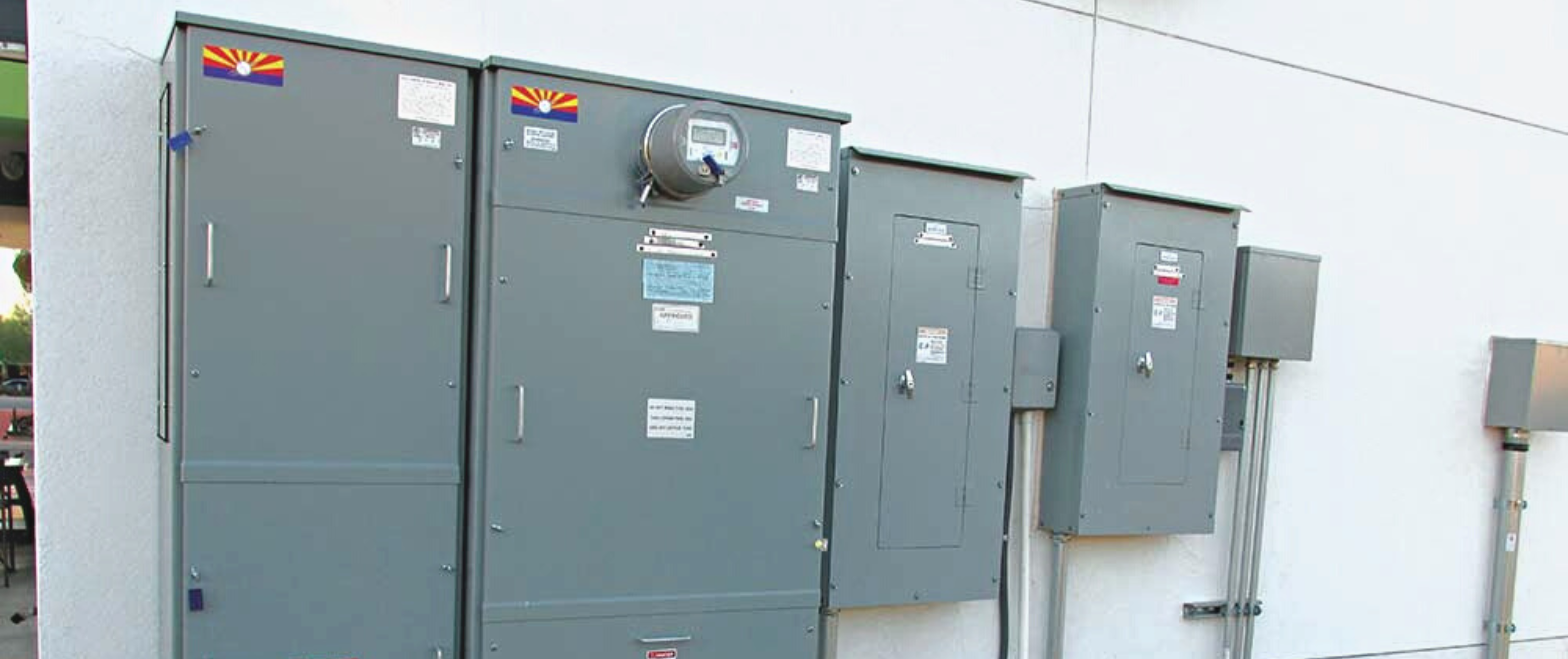# What's the Energy Cost to Run a Heat Treating Kiln?

In commercial settings, electrical heat treating kilns are utilized for a variety of tasks including metallurgy, glass annealing, ceramics and resin curing, just to name a few. Modern kilns use a lot of energy, so figuring out how much energy is being consumed is crucial to figuring out how much it is going to cost to run the unit each time. The cost of operating the unit over a long period can far exceed the purchase price, depending on the unit, So, it's important to know what those operating costs look like. In this article we'll cover the basics for estimating how much energy is consumed by an electrical heat treating kiln.

Most digital controllers allow you to enter your cost per kilowatt hour for electricity and they will report back the cost of the firing.

Things you'll need to know:

Power Rating
The kiln's power rating is the first consideration when estimating energy usage. This is expressed in kW (Kilowatts) or BTUs (British Thermal Units) per hour. Usually, the nameplate of the kiln or the manufacturer's specs will list the power rating. Kilowatt-Hours (kWh) is a standard unit of energy consumption used by the utility company. To calculate the energy used by the kiln in kWh, you need to multiply the kiln's power rating by the duration of the operation in hours. For example, if the kiln is rated at 10 kW and operated for 8 hours, the energy consumption would be approximately 80 kWh. But these's more to it than just this.

Ampere-Hours (Ah)
Another unit of energy consumption that can be used to estimate the energy required by the kiln is ampere-hours (Ah). You must divide the kiln's current rating in amperes by the period of operation in hours to determine the energy consumed in Ah. For instance, if the kiln is rated at 50 amps and is used for 8 hours, 400 Ah of energy will be used.

Things you'll need to Know:

Energy Cost/Price - Depending on the region and the energy source, different energy costs apply. When estimating how much energy the kiln uses, it's critical to take the cost of energy into account. Either the utility company or the energy supplier/provider can provide you with the cost of energy.

Kiln Efficiency - The efficiency of the kiln plays a crucial role in determining energy consumption. A more efficient kiln will require less energy to heat up and  to maintain the desired temperature. The common understanding is that a brick kiln might use more energy to heat up, but less energy to maintain temperature due its refractory material make up. A Fiber kiln will use less energy to heat and cool down, but will need more fuel to maintain its temperature. A kiln's efficiency can be improved by using the appropriate superior materials for the project and ensuring that the kiln is properly sealed so that no heat escapes.

Time and Temperature - And finally, the last variable aspects to take into account are the temperature and length time the kiln is in operation. The kiln/oven/furnace will use more energy as the temperature and operating time increase. To reduce energy consumption and achieve the intended outcome, it is critical to adjust the temperature and duration of the operation.

So here's what it all looks like:

Here’s one formula for calculating the cost of firing your kiln:
1 = Amps

2 = Volts

3 = Estimated Firing time in hours time x .75 (kiln is only on full power
intermittently- meaning you have a slower ramp time, if you are going full power than take out the reduction or adjust that)

4 = Cost per kilowatt hr from your electric bill

1 x 2 x 3 x 4 = Firing Cost 1,000

A longer firing will increase the energy cost, it will not be a large increase because the kiln will not be on full power any longer and the .75 factor in “3” of the formula would be lower. The formula assumes a normal, even increase per hour.

Here’s another way to calculate electric usage and costs:

Step 1: Determine the wattage of the kiln (Volts x Amps = Watts... ex. 120 volts x 15 Amps = 1800 Watts)

Step 2: Multiply wattage by 1000 (ex. 1800 Watts / 1000 = 1.8 KW)

Step 3: Multiply kilowatts (KW) by KW cost on your energy bill (ex. 1.8 KW x \$0.15 = \$0.27 per KWH  for each hour the kiln is on high and not cycling on and off)

Step 4: Multiply the answer you received above by .75 (ex. \$.27 x .75 = \$.20 per hour to run the kiln) You can adjust the reduction percentage or eliminate it if you are going full power to your target temperatures.

Hopefully this covers the basics of calculating costs and what you should consider when doing that task. Most digital controller will allow you to enter your parameters and will report the cost of each firing. Ask your Heatreatnow.com representative for assistance.

As always, feel free to reach out to our experienced team with questions or comments. We're always here to help! Give our technical team a call at (210) 446-9979 or email us at customerservice@heattreatnow.com